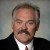# I am gathering attribute data. Sample size is 5 pieces. I collect 20 samples with zero defect. How do I calculate ppm and Cpk?

ByDr. Mikel Harry

Under the condition that D = 0 defects for the constrained condition of N = 20 samples, we can statistically approximate the short-term capability and provide its quantity in the form Z.st = 3.33.  Of course, this is directly equivalent to Cp = 1.11.

Handpicked Content:   Can you explain "degrees of freedom" in the context of a Six Sigma tool, such as a DOE?

Interestingly, the statistical base underpinning this set of computations is fully supported by the 50th percentile of the chi-square distribution.  This is to say there would exist a 50 percent chance that the “true” capability would be lower (or higher) than the given estimate of Z.st = 3.33.  In this sense, it represents the “best guess.”

Given the estimate of instantaneous capability, the defects-per-million opportunities can be ascertained and presented as DPMO = 158,655.  Thus, we have an approximation of the long-term quality expectation, but in the form of a defect metric.

Extending the mathematics of such reasoning, we are able to approximate the lower 95 percent confidence interval as Z.st = 2.5.  Obviously, this is to say there would exist at least 95 percent certainty that the true value of Z.st would be greater than 2.5, given that zero defects were observed over the course of 20 opportunities.

An in-depth discussion of this topic can be found within the reference: Harry, M.J. and Lawson, R.J. (1988). Six Sigma Producibility Analysis and Process Characterization. Publication Number 6S-3-03/88. Motorola University Press, Motorola Inc., Schaumburg Illinois.